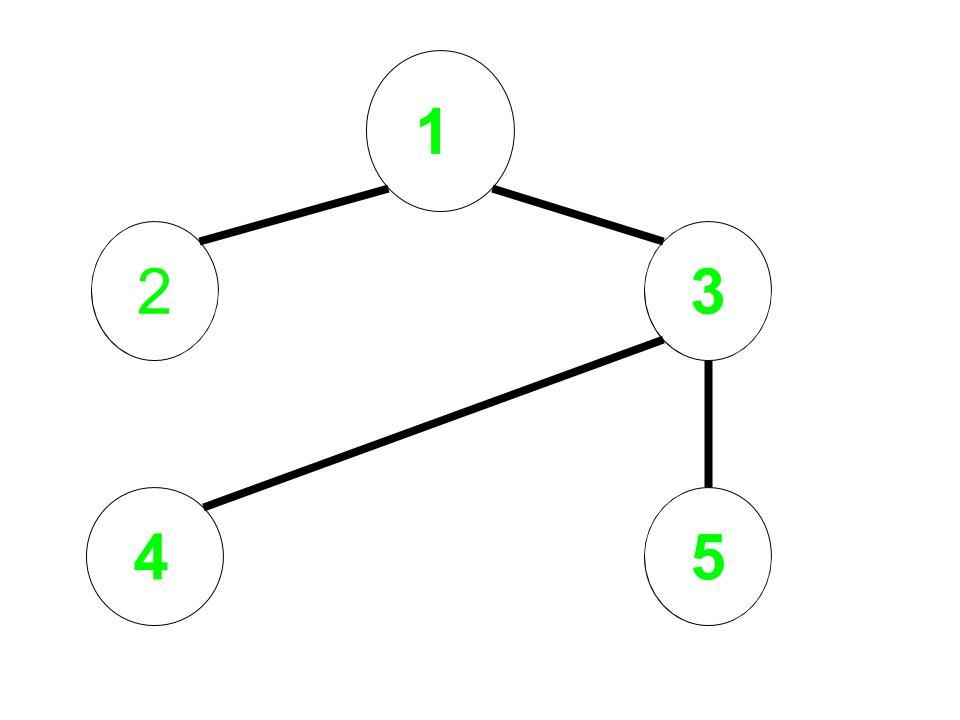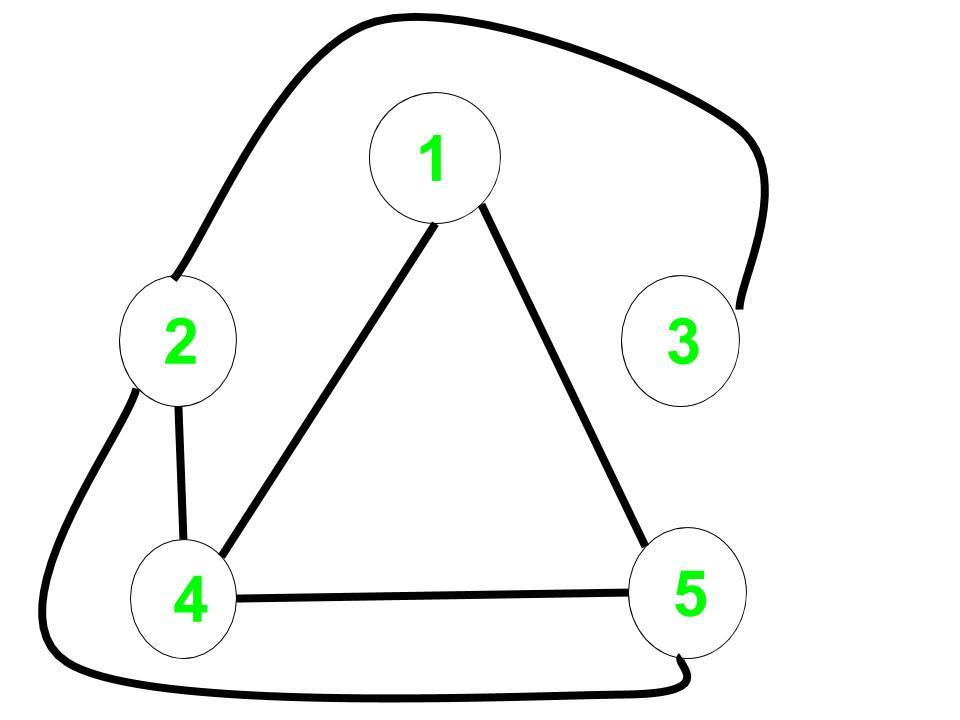# Shortest path in a complement graph

• Difficulty Level : Hard
• Last Updated : 28 Jan, 2021

Given an undirected non-weighted graph G. For a given node start return the shortest path that is the number of edges from start to all the nodes in the complement graph of G.

Complement Graph is a graph such that it contains only those edges which are not present in the original graph.

Examples:

Input: Undirected Edges = (1, 2), (1, 3), (3, 4), (3, 5), Start = 1
Output: 0 2 3 1 1
Explanation:
Original Graph:Complement Graph:The distance from 1 to every node in the complement graph are:
1 to 1 = 0,
1 to 2 = 2,
1 to 3 = 3,
1 to 4 = 1,
1 to 5 = 1

Naive Approach: A Simple solution will be to create the complement graph and use Breadth-First Search on this graph to find the distance to all the nodes.
Time complexity: O(n2) for creating the complement graph and O(n + m) for breadth first search.
Efficient Approach: The idea is to use Modified Breadth-First Search to calculate the answer and then there is no need to construct the complement graph.

• For each vertex or node, reduce the distance of a vertex which is a complement to the current vertex and has not been discovered yet.
• For the problem, we have to observe that if the Graph is Sparse then the undiscovered nodes will be visited very fast.

Below is the implementation of the above approach:

## C++

 `// C++ implementation to find the``// shortest path in a complement graph` `#include ``using` `namespace` `std;` `const` `int` `inf = 100000;` `void` `bfs(``int` `start, ``int` `n, ``int` `m,``         ``map, ``int``> edges)``{``    ``int` `i;` `    ``// List of undiscovered vertices``    ``// initially it will contain all``    ``// the vertices of the graph``    ``set<``int``> undiscovered;` `    ``// Distance will store the distance``    ``// of all vertices from start in the``    ``// complement graph``    ``vector<``int``> distance_node(10000);` `    ``for` `(i = 1; i <= n; i++) {` `        ``// All vertices are undiscovered``        ``undiscovered.insert(i);` `        ``// Let initial distance be infinity``        ``distance_node[i] = inf;``    ``}` `    ``undiscovered.erase(start);``    ``distance_node[start] = 0;``    ``queue<``int``> q;` `    ``q.push(start);` `    ``// Check if queue is not empty and the``    ``// size of undiscovered vertices``    ``// is greater than 0``    ``while` `(undiscovered.size() && !q.empty()) {``        ``int` `cur = q.front();``        ``q.pop();` `        ``// Vector to store all the complement``        ``// vertex to the current vertex``        ``// which has not been``        ``// discovered or visited yet.``        ``vector<``int``> complement_vertex;` `        ``for` `(``int` `x : undiscovered) {``          ` `            ``if` `(edges.count({ cur, x }) == 0 &&``                ``edges.count({ x, cur })==0)``                ``complement_vertex.push_back(x);``        ``}``        ``for` `(``int` `x : complement_vertex) {` `            ``// Check if optimal change``            ``// the distance of this``            ``// complement vertex``            ``if` `(distance_node[x]``                ``> distance_node[cur] + 1) {``                ``distance_node[x]``                    ``= distance_node[cur] + 1;``                ``q.push(x);``            ``}` `            ``// Finally this vertex has been``            ``// discovered so erase it from``            ``// undiscovered vertices list``            ``undiscovered.erase(x);``        ``}``    ``}``    ``// Print the result``    ``for` `(``int` `i = 1; i <= n; i++)``        ``cout << distance_node[i] << ``" "``;``}` `// Driver code``int` `main()``{``    ``// n is the number of vertex``    ``// m is the number of edges``    ``// start - starting vertex is 1``    ``int` `n = 5, m = 4;` `    ``// Using edge hashing makes the``    ``// algorithm faster and we can``    ``// avoid the use of adjacency``    ``// list representation``    ``map, ``int``> edges;` `    ``// Initial edges for``    ``// the original graph``    ``edges[{ 1, 3 }] = 1,``                 ``edges[{ 3, 1 }] = 1;``    ``edges[{ 1, 2 }] = 1,``                 ``edges[{ 2, 1 }] = 1;``    ``edges[{ 3, 4 }] = 1,``                 ``edges[{ 4, 3 }] = 1;``    ``edges[{ 3, 5 }] = 1,``                 ``edges[{ 5, 3 }] = 1;` `    ``bfs(1, n, m, edges);` `    ``return` `0;``}`

## Java

 `// Java implementation to find the``// shortest path in a complement graph``import` `java.io.*;``import` `java.util.*;` `class` `GFG{``    ` `// Pair class is made so as to``// store the edges between nodes``static` `class` `Pair``{``    ``int` `left;``    ``int` `right;``    ` `    ``public` `Pair(``int` `left, ``int` `right)``    ``{``        ``this``.left = left;``        ``this``.right = right;``    ``}``    ` `    ``// We need to override hashCode so that``    ``// we can use Set's properties like contains()``    ``@Override``    ``public` `int` `hashCode()``    ``{``        ``final` `int` `prime = ``31``;``        ``int` `result = ``1``;``        ``result = prime * result + left;``        ``result = prime * result + right;``        ``return` `result;``    ``}` `    ``@Override``    ``public` `boolean` `equals( Object other )``    ``{``        ``if` `(``this` `== other){``return` `true``;}``        ``if` `(other ``instanceof` `Pair)``        ``{``            ``Pair m = (Pair)other;``            ``return` `this``.left == m.left &&``                  ``this``.right == m.right;``        ``}``        ``return` `false``;``    ``}``}` `public` `static` `void` `bfs(``int` `start, ``int` `n, ``int` `m,``                       ``Set edges)``{``    ``int` `i;` `    ``// List of undiscovered vertices``    ``// initially it will contain all``    ``// the vertices of the graph``    ``Set undiscovered = ``new` `HashSet<>();` `    ``// Distance will store the distance``    ``// of all vertices from start in the``    ``// complement graph``    ``int``[] distance_node = ``new` `int``[``1000``];` `    ``for``(i = ``1``; i <= n; i++)``    ``{` `        ``// All vertices are undiscovered initially``        ``undiscovered.add(i);` `        ``// Let initial distance be maximum value``        ``distance_node[i] = Integer.MAX_VALUE;``    ``}``    ` `    ``// Start is discovered``    ``undiscovered.remove(start);``    ` `    ``// Distance of the node to itself is 0``    ``distance_node[start] = ``0``;``    ` `    ``// Queue used for BFS``    ``Queue q = ``new` `LinkedList<>();` `    ``q.add(start);` `    ``// Check if queue is not empty and the``    ``// size of undiscovered vertices``    ``// is greater than 0``    ``while` `(undiscovered.size() > ``0` `&& !q.isEmpty())``    ``{``        ` `        ``// Current node``        ``int` `cur = q.peek();``        ``q.remove();` `        ``// Vector to store all the complement``        ``// vertex to the current vertex``        ``// which has not been``        ``// discovered or visited yet.``        ``Listcomplement_vertex = ``new` `ArrayList<>();` `        ``for``(``int` `x : undiscovered)``        ``{``            ``Pair temp1 = ``new` `Pair(cur, x);``            ``Pair temp2 = ``new` `Pair(x, cur);``            ` `            ``// Add the edge if not already present``            ``if` `(!edges.contains(temp1) &&``                ``!edges.contains(temp2))``            ``{``                ``complement_vertex.add(x);``            ``}``        ``}``        ` `        ``for``(``int` `x : complement_vertex)``        ``{``            ` `            ``// Check if optimal change``            ``// the distance of this``            ``// complement vertex``            ``if` `(distance_node[x] >``                ``distance_node[cur] + ``1``)``            ``{``                ``distance_node[x] =``                ``distance_node[cur] + ``1``;``                ``q.add(x);``            ``}` `            ``// Finally this vertex has been``            ``// discovered so erase it from``            ``// undiscovered vertices list``            ``undiscovered.remove(x);``        ``}``    ``}``    ` `    ``// Print the result``    ``for``(i = ``1``; i <= n; i++)``        ``System.out.print(distance_node[i] + ``" "``);``}``    ` `// Driver code``public` `static` `void` `main(String[] args)``{``    ` `    ``// n is the number of vertex``    ``// m is the number of edges``    ``// start - starting vertex is 1``    ``int` `n = ``5``, m = ``4``;` `    ``// Using edge hashing makes the``    ``// algorithm faster and we can``    ``// avoid the use of adjacency``    ``// list representation``    ``Set edges = ``new` `HashSet<>();` `    ``// Initial edges for``    ``// the original graph``    ``edges.add(``new` `Pair(``1``, ``3``));``    ``edges.add(``new` `Pair(``3``, ``1``));``    ``edges.add(``new` `Pair(``1``, ``2``));``    ``edges.add(``new` `Pair(``2``, ``1``));``    ``edges.add(``new` `Pair(``3``, ``4``));``    ``edges.add(``new` `Pair(``4``, ``3``));``    ``edges.add(``new` `Pair(``3``, ``5``)) ;``    ``edges.add(``new` `Pair(``5``, ``3``));``    ``Pair t = ``new` `Pair(``1``, ``3``);` `    ``bfs(``1``, n, m, edges);``}``}` `// This code is contributed by kunalsg18elec`

Output:

`0 2 3 1 1`

Time complexity: O(V+E)
Auxiliary Space: O(V)

My Personal Notes arrow_drop_up